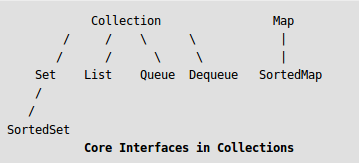# Sort elements by frequency | Set 5 (using Java Map)

Given an integer array, sort the array according to the frequency of elements in decreasing order, if frequency of two elements are same then sort in increasing order

Examples:

```Input: arr[] = {2, 3, 2, 4, 5, 12, 2, 3, 3, 3, 12}
Output: 3 3 3 3 2 2 2 12 12 4 5
Explanation :
No. Freq
2  : 3
3  : 4
4  : 1
5  : 1
12 : 2

Input: arr[] = {4, 4, 2, 2, 2, 2, 3, 3, 1, 1, 6, 7, 5}
Output: 2 2 2 2 1 1 3 3 4 4 5 6 7
```

## Recommended: Please solve it on “PRACTICE” first, before moving on to the solution.

Different approaches have been discussed in below posts:
Sort elements by frequency | Set 1
Sort elements by frequency | Set 2
Sorting Array Elements By Frequency | Set 3 (Using STL)
Sort elements by frequency | Set 4 (Efficient approach using hash)

Approach:

Java Map has been used in this set to solve the problem. The java.util.Map interface represents a mapping between a key and a value. The Map interface is not a subtype of the Collection interface. Therefore it behaves a bit different from the rest of the collection types.In the below program:

• Get the element with its count in a Map
• By using the Comparator Interface, compare the frequency of an elements in a given list.
• Use this comparator to sort the list by implementing Collections.sort() method.
• Print the sorted list.

Program:

 `import` `java.util.*; ` ` `  `public` `class` `GFG { ` ` `  `    ``// Driver Code ` `    ``public` `static` `void` `main(String[] args) ` `    ``{ ` ` `  `        ``// Declare and Initialize an array ` `        ``int``[] array = { ``4``, ``4``, ``2``, ``2``, ``2``, ``2``, ``3``, ``3``, ``1``, ``1``, ``6``, ``7``, ``5` `}; ` ` `  `        ``Map map = ``new` `HashMap<>(); ` `        ``List outputArray = ``new` `ArrayList<>(); ` ` `  `        ``// Assign elements and their count in the list and map ` `        ``for` `(``int` `current : array) { ` `            ``int` `count = map.getOrDefault(current, ``0``); ` `            ``map.put(current, count + ``1``); ` `            ``outputArray.add(current); ` `        ``} ` ` `  `        ``// Compare the map by value ` `        ``SortComparator comp = ``new` `SortComparator(map); ` ` `  `        ``// Sort the map using Collections CLass ` `        ``Collections.sort(outputArray, comp); ` ` `  `        ``// Final Output ` `        ``for` `(Integer i : outputArray) { ` `            ``System.out.print(i + ``" "``); ` `        ``} ` `    ``} ` `} ` ` `  `// Implement Comparator Interface to sort the values ` `class` `SortComparator ``implements` `Comparator { ` `    ``private` `final` `Map freqMap; ` ` `  `    ``// Assign the specified map ` `    ``SortComparator(Map tFreqMap) ` `    ``{ ` `        ``this``.freqMap = tFreqMap; ` `    ``} ` ` `  `    ``// Compare the values ` `    ``@Override` `    ``public` `int` `compare(Integer k1, Integer k2) ` `    ``{ ` ` `  `        ``// Compare value by frequency ` `        ``int` `freqCompare = freqMap.get(k2).compareTo(freqMap.get(k1)); ` ` `  `        ``// Compare value if frequency is equal ` `        ``int` `valueCompare = k1.compareTo(k2); ` ` `  `        ``// If frequency is equal, then just compare by value, otherwise - ` `        ``// compare by the frequency. ` `        ``if` `(freqCompare == ``0``) ` `            ``return` `valueCompare; ` `        ``else` `            ``return` `freqCompare; ` `    ``} ` `} `

Output:

```2 2 2 2 1 1 3 3 4 4 5 6 7
```

Time Complexity : O(n Log n)

My Personal Notes arrow_drop_upCheck out this Author's contributed articles.

If you like GeeksforGeeks and would like to contribute, you can also write an article using contribute.geeksforgeeks.org or mail your article to contribute@geeksforgeeks.org. See your article appearing on the GeeksforGeeks main page and help other Geeks.

Please Improve this article if you find anything incorrect by clicking on the "Improve Article" button below.

Practice Tags :

6

Please write to us at contribute@geeksforgeeks.org to report any issue with the above content.